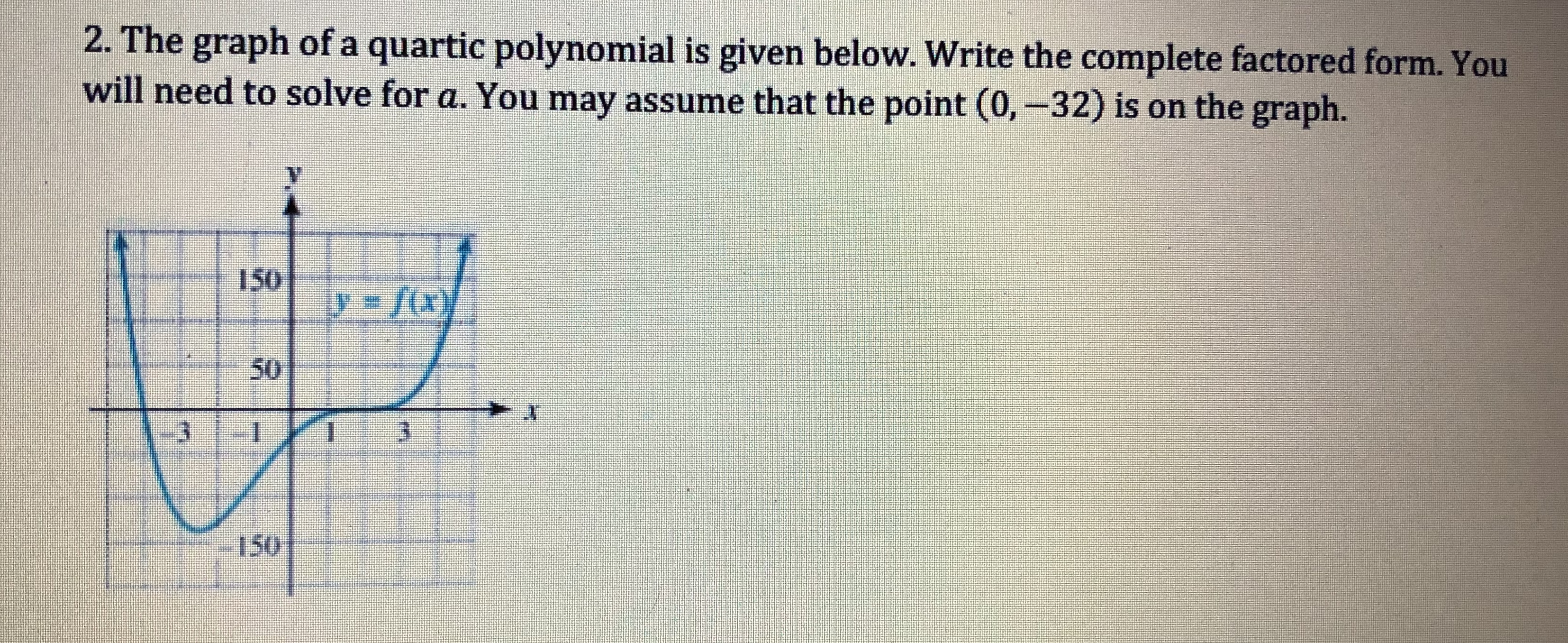2. The graph of a quartic polynomial is given below. Write the complete factored form. Youwill need to solve for a. You may assume that the point (0-32) is on the graph.

Questionhelp_outlineImage Transcriptionclose2. The graph of a quartic polynomial is given below. Write the complete factored form. You will need to solve for a. You may assume that the point (0-32) is on the graph. fullscreen
Step 1

Given that y = f(x) is a quartic polynomial. So it has degree 4 and it pass through point (0,-32), which is the y intercept.

Here we can see that if x tends to ∞ then f(x) tends to ∞ and x tends to - then f(x) tends to ∞. So we can say that a is a positive number since the degree of polynomial is 4 which is even.

Step 2

We can see that the graph cross x axis at x = -3 and at x = 2 and we know the multiplicity of zero of f(x) a...

Want to see the full answer?

See Solution

Want to see this answer and more?

Our solutions are written by experts, many with advanced degrees, and available 24/7

See Solution
Tagged in

Polynomials